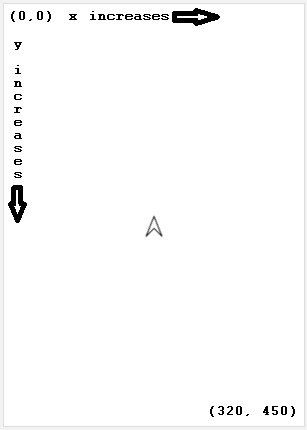# move

Category:Turtle

## move(x, y)

Category: Turtle

Moves the turtle by adding x pixels to the turtle's current x position and y pixels to the turtle's current y position.

Some drawings are more easily described by movements of the turtle relative to the current turtle location. Unlike moveForward(pixels), the turtle can move in a direction different from its facing direction.

### Examples

```// Draw a line while moving the turtle to the right and down.
move(50, 50);
```

Example: Turtle Direction Irrelevant Draw the same line as the previous example but with the turtle facing a different direction.

```// Draw the same line as the previous example but with the turtle facing a different direction.
turnRight(90);
move(50, 50);
```

Example: Arrow Draw a arrow pointing up from a random location on the screen.

 ```// Draw a arrow pointing up from a random location on the screen. penUp(); moveTo(randomNumber(320),randomNumber(480)); penDown(); move(0, -100); move(-25, 50); move(50, 0); move(-25, -50); ```### Syntax

```move(x, y);
```

### Parameters

Name Type Required? Description
x number Yes The number of pixels to move the turtle right.
y number Yes The number of pixels to move the turtle down.

### Returns

No return value. Moves turtle only.

### Tips

• Use penUp() before calling move(x,y) to have the turtle not draw as it moves.
• The screen default size is 320 pixels wide and 450 pixels high, but you can move the turtle off the screen by exceeding those dimensions.
• There are three ways to move the turtle in a straight line:
• Specify the number of pixels to move the turtle in the direction it is facing using moveForward(pixels) or moveBackward(pixels).
• Specify a number of pixels in the x and y direction to move the turtle using move(x,y), regardless of direction that the turtle is facing.
• Specify an x and y pixel location on the screen to move the turtle to using moveTo(x,y), regardless of direction that the turtle is facing.Found a bug in the documentation? Let us know at documentation@code.org

Found a bug in the documentation? Let us know at documentation@code.org